# Quadric

(diff) ← Older revision | Latest revision (diff) | Newer revision → (diff)
Jump to: navigation, search

A quadric is a surface of the second order. In a three-dimensional (projective, affine or Euclidean) space a quadric is a set of points whose homogeneous coordinates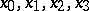(with respect to a projective, affine or Cartesian system of coordinates) satisfy a homogeneous equation of degree two:The bilinear symmetric formis called the polar form relative to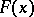. Two points,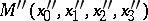at which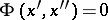are called conjugate points with respect to the quadric. If the line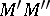intersects the quadric at the points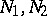and the points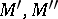are conjugate to each other with respect to the quadric, thenandform a harmonic quadruple. The points of a quadric and only these are self-conjugate. A line all points of which lie on a quadric is called a generator of the quadric. By the pole of a given plane with respect to a quadric is meant the point that is conjugate to every point of this plane. The set of points in the space that are conjugate to a given point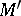with respect to a quadric is called the polar ofwith respect to the quadric. A tangent plane to a quadric is the polar of the point of contact. The polar of a pointis defined by the linear equation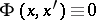with respect to the coordinates. If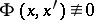, then the polar ofis a plane; if, then the polar ofis the whole space. In this casebelongs to the quadric and is called a singular point of it. If, then the quadric has no singular points and is called a non-degenerate quadric. In projective space this is an imaginary ovaloid, a real ovaloid or a ruled quadric. A non-degenerate quadric determines a correlation (or polarity), i.e. a bijective mapping from the set of points of projective space onto the set of planes. A ruled non-degenerate quadric has two distinct families of generators, distributed on the quadric so that any two lines of the same family are non-intersecting, while two lines of different families intersect at one point. If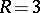, then a quadric is a cone (real or imaginary) with vertex at the unique singular point. A real cone has a single family of generators, passing through its vertex. If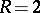, then the quadric splits into a pair of planes (real or imaginary), intersecting in a line consisting of its singular points. If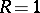, then a quadric is a double real plane consisting of singular points. The affine properties of a quadric are distinguished by its behaviour with respect to the plane at infinity,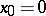. For example, an ellipsoid (hyperboloid, paraboloid) is a non-degenerate quadric that does not intersect (does intersect, is tangent to) the plane at infinity. The centre of a quadric is the pole of the plane at infinity, a diameter is a line through the centre.

## Contents

How to Cite This Entry:
Quadric. Encyclopedia of Mathematics. URL: http://encyclopediaofmath.org/index.php?title=Quadric&oldid=11769
This article was adapted from an original article by V.S. Malakhovskii (originator), which appeared in Encyclopedia of Mathematics - ISBN 1402006098. See original article